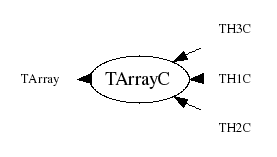library: libCore#include "TArrayC.h"

# TArrayC

class description - header file - source file

## class TArrayC: public TArray### Function Members (Methods)

Display options:
Show inherited
Show non-public
public:
 TArrayC() TArrayC(Int_t n) TArrayC(const TArrayC& array) TArrayC(Int_t n, const Char_t* array) virtual ~TArrayC() void AddAt(Char_t c, Int_t i) void Adopt(Int_t n, Char_t* array) Char_t At(Int_t i) const static TClass* Class() void Copy(TArrayC& array) const const Char_t* GetArray() const Char_t* GetArray() Int_t TArray::GetSize() const Stat_t GetSum() const virtual TClass* IsA() const TArrayC& operator=(const TArrayC& rhs) Char_t& operator[](Int_t i) Char_t operator[](Int_t i) const static TArray* TArray::ReadArray(TBuffer& b, const TClass* clReq) void Reset(Char_t val = 0) virtual void Set(Int_t n) void Set(Int_t n, const Char_t* array) virtual void ShowMembers(TMemberInspector& insp, char* parent) virtual void Streamer(TBuffer& b) void StreamerNVirtual(TBuffer& b) static void TArray::WriteArray(TBuffer& b, const TArray* a)
protected:
 Bool_t TArray::BoundsOk(const char* where, Int_t at) const Bool_t TArray::OutOfBoundsError(const char* where, Int_t i) const

### Data Members

public:
 Char_t* fArray [fN] Array of fN chars Int_t TArray::fN Number of array elements

## Class Description

```
TArrayC

Array of chars or bytes (8 bits per element).

```
TArrayC()
``` Default TArrayC ctor.
```
TArrayC(Int_t n)
``` Create TArrayC object and set array size to n chars.
```
TArrayC(Int_t n, const Char_t *array)
``` Create TArrayC object and initialize it with values of array.
```
TArrayC(const TArrayC &array)
``` Copy constructor.
```
TArrayC & operator=(const TArrayC &rhs)
``` TArrayC assignment operator.
```
~TArrayC()
``` Delete TArrayC object.
```
``` Adopt array arr into TArrayC, i.e. don't copy arr but use it directly
in TArrayC. User may not delete arr, TArrayC dtor will do it.
```
``` Add char c at position i. Check for out of bounds.
```
void Set(Int_t n)
``` Set size of this array to n chars.
A new array is created, the old contents copied to the new array,
then the old array is deleted.
This function should not be called if the array was declared via Adopt.
```
void Set(Int_t n, const Char_t *array)
``` Set size of this array to n chars and set the contents.
This function should not be called if the array was declared via Adopt.
```
void Streamer(TBuffer &b)
``` Stream a TArrayC object.
```

void Copy(TArrayC &array)
`{array.Set(fN,fArray);}`
const Char_t * GetArray()
`{ return fArray; }`

`{ return fArray; }`

`{Stat_t sum=0; for (Int_t i=0;i<fN;i++) sum+=fArray[i]; return sum;}`
void Reset(Char_t val=0)
`{memset(fArray,val,fN*sizeof(Char_t));}`

Author: Rene Brun 06/03/95
Last update: root/cont:\$Name: \$:\$Id: TArrayC.cxx,v 1.14 2005/11/16 20:07:50 pcanal Exp \$